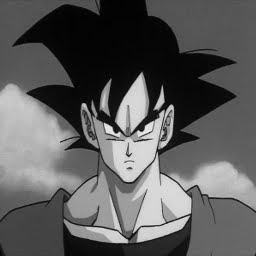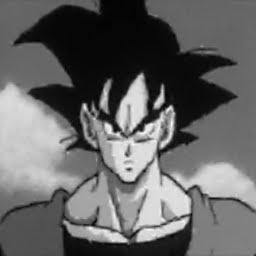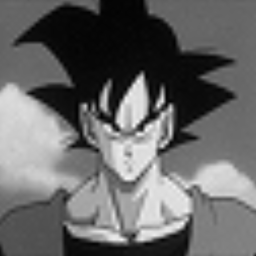## Monday, 21 August 2017

### Spatially biased sampling with revector fields: Proof of concept

tldr: A uv coordinate transform field can transform texture sampling into something akin to a 2d quad mesh. This allows for much better representations of 2d vector graphics when represented as textures.

### Sampling

Optimal sampling of raw spatial data does not necessarily consist of uniformly sampled points. While sampling at twice the lowest frequency (Shannon) results in detection of a given frequency the  representation of this data is quite poor (specifically perceptually).

The two figures below compare 1d sin function with a uniform vs spatially biased sampling.
Red lines indicate linearly interpolated sample points. Green bars indicate uniform sampling locations.The figure below shows a 1d sin( x^4) function that exhibits geometric structure.Non-uniform sampling is favorable when either:
1. Geometric structural regularity of data is non uniform
2. Optimal Sampling phase does not match uniform phase

#### Experiment

I decided the best way to demonstrate biased sampling was with 2d sampling aka textures. All sampling of these textures was using bilinear filtering. The two criteria mentioned above apply to cartoon images which is what we consider below.

.Here we have a 256x256 image and its least squared error 64x64 approximation. We introduce a biasing vector field texture (x,y) to transform uniform samples to biased samples.

In pseudo hlsl

Normal sampling:
rgb = texture2d(downsampled64.png,  uv.xy);

Biased revector sampling:
vec2 biasedUV =  texture2d( biasVectorField64 , uv.xy ).xy;
rgb = texture2d( rebiasedDownsampled64 , biasedUV );

I did not have time to develop an algorithm to find both the forward biased vector field and the complementary rebiasedDownsample. So I used stochastic sampling to find both these this field and sampling data. Below is a comparison to the original normal bilinear filtered image to a biased sampled image/field.Obviously this single forward bias field adds an extra texture lookup and the memory and bandwidth of sampling this field texture. (such sampling is coherent however)Radical differences become even more obvious in with magnified portions of these images. The biased vector field has spared no expense to in its attempts at representing the original image with spatially distributed bilinear filtering.

It should be noted that the final results here are only a proof of concept. The results were better than I expected especially considering the stochastic method for generating the solutions.

#### References

Cartoon image: https://en.wikipedia.org/wiki/Goku
Similar work: http://www.valvesoftware.com/publications/2007/SIGGRAPH2007_AlphaTestedMagnification.pdf

Here are some more examples. (With animated stochastic error reduction process)Penguin LogoBasic text.DBZ goku.

#### 1 comment:

1.Hi Peter, with many apologies, no published email address exists for you, I saw your comment on ycombinator, would love to discuss it with you however comments are closed. If you'd like to get in touch my email is luke.leighton@gmail.com